# Boundary Nodes, Elements and Edges: complex mesh boundaries

When the geometry of a mesh is not regular or its boundary is decomposed of many different parts, we can use the edges of the mesh to find the nodes that belongs to the boundary.
The edges corresponding to the boundary are the only ones that belongs only to a unique element. The internal ones are always sheared by two elements. Using this property, we have build the boundaryNodes function (at the end of this document) to find all the nodes and all the edges belonging to the boundary.

## Example:

eval('meshDataHoleS5');
[numElem, ndim] = size(elem);
figure()
plotElements(nodes, elem, 0);
[indNodBd, indElemBd, indLocalEdgBd, edges] = boundaryNodes(nodes, elem);
hold on;
for i=1:length(indElemBd)
fill(nodes(elem(indElemBd(i),:),1),nodes(elem(indElemBd(i),:),2),'y')
end
plot(nodes(indNodBd,1), nodes(indNodBd,2),'rs');
hold off;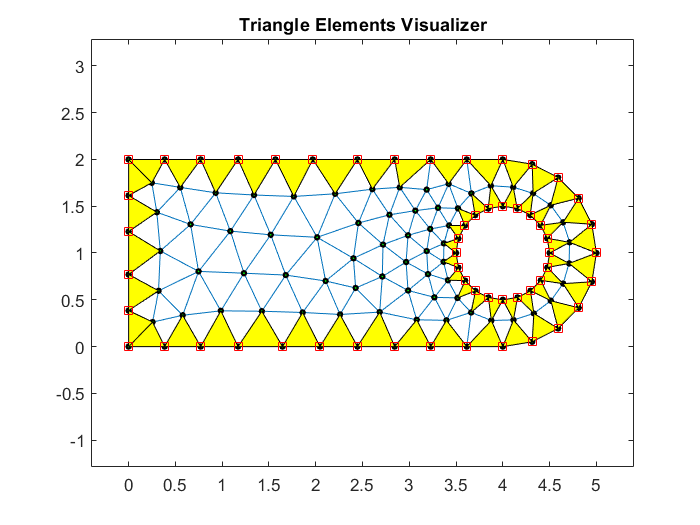## Plot only the corresponding edges of the boundary elements

figure()
for i=1:length(indElemBd)
k1=indLocalEdgBd(i);
k2=k1+1;
if (ndim==3)
if (k1==3)
k2=1;
end
elseif(ndim==4)
if (k1==4)
k2=1;
end
end
v1=nodes(elem(indElemBd(i),k1),:);
v2=nodes(elem(indElemBd(i),k2),:);
X=[v1(1); v2(1)];
Y=[v1(2); v2(2)];
plot(X,Y,'m')
hold on;
end
axis equal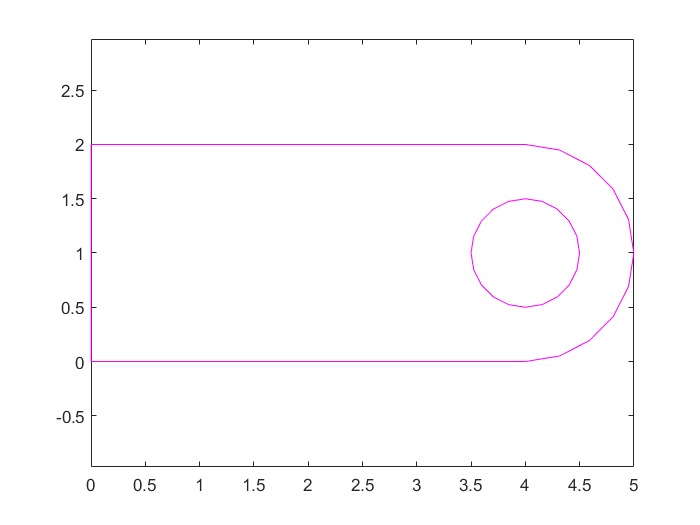## Only one part of the boundary

We can mix the find function together with boundaryNodes function to obtain complementary boundaries using setdiff.
Example: Let's assume that at the boundary we have the same BC and it is only different on the left side of the boundary and in the circle.
We must have three different node list: indLeft, indCircle and indEqual (all the rest).
indLeft = find(nodes(:,1) < 0.001);
indCircle = find(((nodes(:,1)-4).^2+(nodes(:,2)-1).^2 - 0.5^2) < 0.01);
indEqual = setdiff(indNodBd,indLeft); %substract left nodes from all boundary nodes
indEqual = setdiff(indEqual,indCircle); %now substract circle nodes
figure()
plot(nodes(indLeft,1),nodes(indLeft,2),'ro'); %plot on the previous figure
hold on;
plot(nodes(indCircle,1),nodes(indCircle,2),'bo');
plot(nodes(indEqual,1),nodes(indEqual,2),'gs');
axis equal
hold off;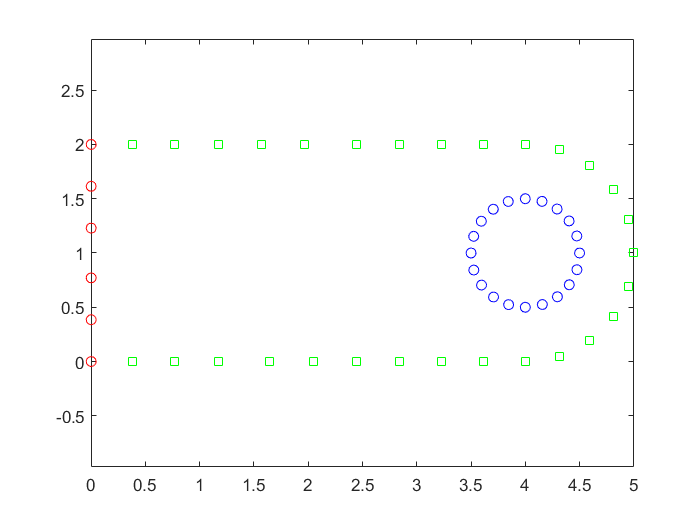## Exercise 1: (easy)

From the mesh file meshUTriang.m and using find and boundaryNodes, select all the boundary nodes according to the figure: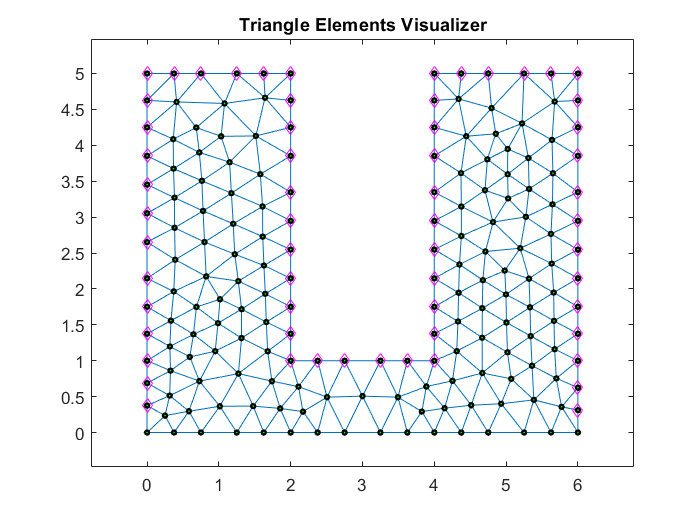Hint: Total number of nodes in the complete boundary 76. Final number of selected nodes 60.

## Exercise 2: (middle)

From the mesh file meshUTriang.m and using find and boundaryNodes, select all the boundary nodes according to the figure: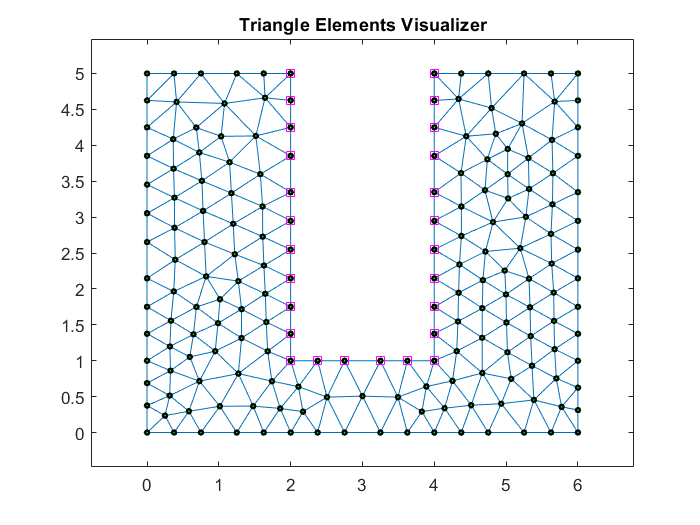Hint: Final number of selected nodes 26.

## Exercise 3: (difficult)

From the mesh file meshClipQuad.m and using find and boundaryNodes, select all the boundary nodes according to the figure: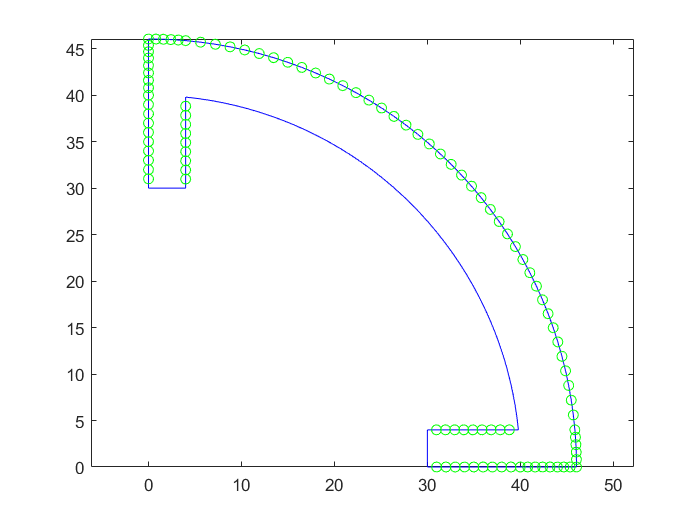## function boundaryNodes: All nodes belonging to the boundary

function [indNodBd, indElemBd, indLocalEdgBd, edges] = boundaryNodes(nodes, elem)
% output:
% indNodBd --> list of all nodes on the boundary
% indElemBd --> list of indices of the Elements on the boundary
% indLocalEdgBd --> list of indices of the local element edges on the boundary
% edges --> list of all edges defined in the total mesh
%
numNod = size(nodes,1);
[numElem, ndim] = size(elem);
if (ndim == 3) %triangle edges
edges = unique(sort([elem(:,[1,2]);elem(:,[2,3]);elem(:,[3,1])],2),'rows');
elseif (ndim == 4) %quadrilateral edges
edges = unique(sort([elem(:,[1,2]);elem(:,[2,3]);elem(:,[3,4]);elem(:,[4,1])],2),'rows');
end
indNodBd=[];
indLocalEdgBd=[];
indElemBd=[];
% look for the edges belonging only to one element
for i=1:size(edges,1)
n1=edges(i,1);
n2=edges(i,2);
[indRow,indCol]=find(elem == n1); %find elements owning the first node
[indElem,col]=find(elem(indRow,:) == n2); % owing also the second one
if (length(indElem) == 1) %boundary edges
indNodBd=[indNodBd; n1; n2];
indElemBd=[indElemBd; indRow(indElem)];
lloc1=find(elem(indRow(indElem),:)==n1);
lloc2=find(elem(indRow(indElem),:)==n2);
if (ndim == 3) %triangle edges
aux=[0,0,0];
aux(lloc1)=1;
aux(lloc2)=1;
number = aux(1)+2*aux(2)+4*aux(3);
switch (number) %identify the appropriate element edge
case 3
edgeBd=1;
case 5
edgeBd=3;
case 6
edgeBd=2;
otherwise, error('edge not allowed');
end
elseif (ndim == 4) %quadrilateral edges
aux=[0,0,0,0];
aux(lloc1)=1;
aux(lloc2)=1;
number = aux(1)+2*aux(2)+4*aux(3)+8*aux(4);
switch (number) %identify the appropriate element edge
case 3
edgeBd=1;
case 6
edgeBd=2;
case 9
edgeBd=4;
case 12
edgeBd=3;
otherwise, error('edge not allowed');
end
end
indLocalEdgBd=[indLocalEdgBd; edgeBd];
end
end
indNodBd=unique(indNodBd);
end
(c)Numerical Factory 2020